# C语言 const

const是一个C语言的关键字，它限定一个变量不允许被改变。使用const在一定程度上可以提高程序的健壮性，另外，在观看别人代码的时候，清晰理解const所起的作用，对理解对方的程序也有一些帮助。

## const只读变量

• const修饰的变量是只读的，本质还是变量
• const修饰的局部变量在上分配空间
• const修饰的全局变量在只读存储区分配空间
• const只在编译期有用，在运行期无用

const修饰的变量不是真的常量，它只是告诉编译器该变量不能出现在赋值符号的左边

## const修饰全局变量

### 示例：const变量的本质

``````#include <stdio.h>

const int g_cc = 2;

int main()
{
const int cc = 1;

int* p = (int*)&cc;

printf("cc = %d\n", cc);

*p = 3;

printf("cc = %d\n", cc);

p = (int*)&g_cc;

printf("g_cc = %d\n", g_cc);

*p = 4;

printf("g_cc = %d\n", g_cc);

return 0;
}
``````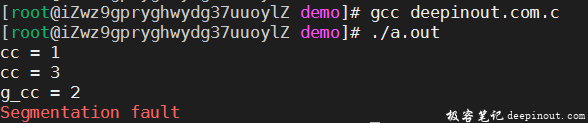• const修饰的局部变量的值被改变了。
• const修饰的全局变量，被存放在只读存储区，修改其值时发生了segmentation fault。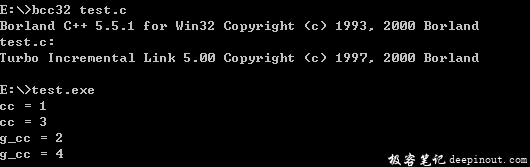## const的本质

C 语言中的const 使得变量具有只读属性。

### 示例： const的本质分析

``````#include <stdio.h>

const int g_array = {0};

void modify(int* p, int v)
{
*p = v;
}

int main()
{
int const i = 0;
const static int j = 0;
int const array = {0};

modify((int*)&i, 1);           // ok
modify((int*)&j, 2);           // error, j被存放于只读存储区，不能被修改
modify((int*)&array, 3);    // ok
modify((int*)&g_array, 4);  // error，g_array被存放于只读存储区，不能被修改

printf("i = %d\n", i);
printf("j = %d\n", j);
printf("array = %d\n", array);
printf("g_array = %d\n", g_array);

return 0;
}
``````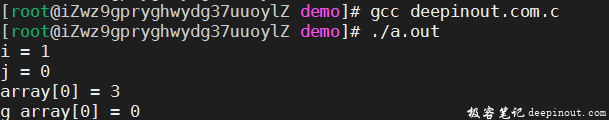## const修饰函数参数和返回值

const修饰函数参数表示在函数体内不希望改变参数的值，const修饰函数返回值表示返回值不可改变，多用于返回指针的情况。

C语言中的字符串字面量存储于只读存储区中，在程序中需要使用`const char*`指针。

``````#include<stdio.h>

int main(){
const char *s = "www.deepinout.com";//字符串字面量
return 0;
}
``````

### 示例：const 修饰函数参数和返回值

``````#include <stdio.h>

const char* f(const int i)
{
i = 5; //error, i被const修饰，在函数体内部不能被改变，不能成为左值

return "deepinout.com";
}

int main()
{
char* pc = f(0); // const char* pc = f(0);

printf("%s\n", pc);

pc = '_';// f函数返回的只读存储区的内容，这里修改会报错

printf("%s\n", pc);

return 0;
}
``````

gcc编译结果：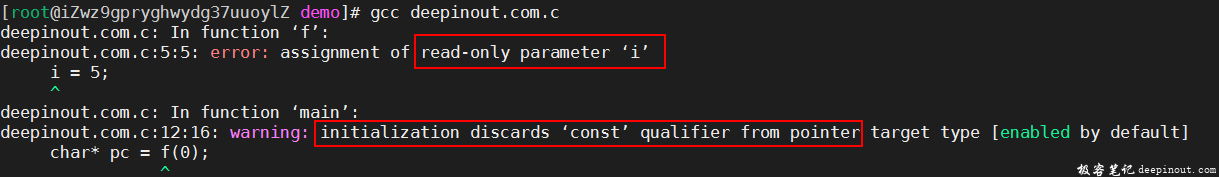## const总结

• const使得变量具有只读属性
• const不能定义真正意义上的常量
• const将具有全局生命周期的变量存储于只读存储区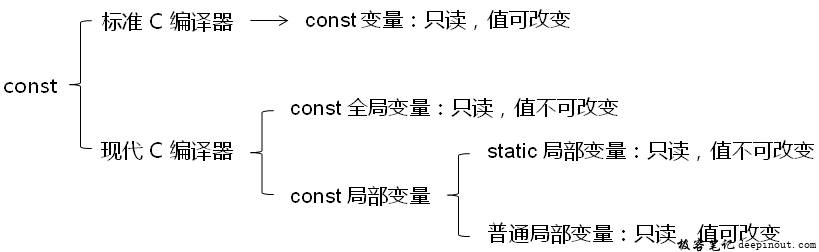• 回顶